### Example 5.5 Multiple Subproblems

Many important optimization problems cannot be solved directly using a standard solver, either because the problem has constraints that cannot be modeled directly or because the resulting model would be too large to be practical. For these types of problems, you can use PROC OPTMODEL to synthesize solution methods by using a combination of the existing solvers and the modeling language programming constructions. This example demonstrates the use of multiple subproblems to solve the cutting stock problem.

The cutting stock problem determines how to efficiently cut raw stock into finished widths based on the demands for the final product. Consider the example from page 195 of Chvátal (1983), where raw stock has a width of 100 inches and the demands are shown in Table 5.15.

Table 5.15: Cutting Stock Demand

 Finished Width Demand 45 inches 97 35 inches 610 31 inches 395 14 inches 211

A portion of the demand can be satisfied using a cutting pattern. For example, with the demands in Table 5.15 a possible pattern cuts one final of width 35 inches, one final of width 31 inches, and two finals of width 14 inches. This gives: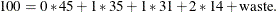The cutting stock problem can be formulated as follows, where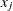represents the number of times patternappears,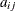represents the number of times demand itemappears in pattern,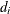is the demand for item,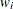is the width of item,
represents the set of patterns,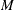represents the set of items, and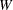is the width of the raw stock: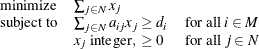Also for each feasible patternyou must have: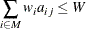The difficulty with this formulation is that the number of patterns can be very large, with too many columnsto solve efficiently. But you can use column generation, as described on page 198 of Chvátal (1983), to generate a smaller set of useful patterns, starting from an initial feasible set.

The dual variables,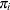, of the demand constraints are used to price out the columns. From linear programming (LP) duality theory, a column that improves the primal solution must have a negative reduced cost. For this problem the reduced cost for columnis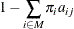Using this observation produces a knapsack subproblem: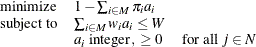where the objective is equivalent to: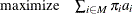The pattern is useful if the associated reduced cost is negative: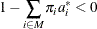So you can use the following steps to generate the patterns and solve the cutting stock problem:

1. Initialize a set of trivial (one item) patterns.

2. Solve the problem using the LP solver.

3. Minimize the reduced cost using a knapsack solver.

4. Include the new pattern if the reduced cost is negative.

5. Repeat steps 2 through 4 until there are no more negative reduced cost patterns.

These steps are implemented in the following statements. Since adding columns preserves primal feasibility, the statements use the primal simplex solver to take advantage of a warm start. The statements also solve the LP relaxation of the problem, but you want the integer solution. So the statements finish by using the MILP solver with the integer restriction applied. The result is not guaranteed to be optimal, but lower and upper bounds can be provided for the optimal objective.

/* cutting-stock problem */
/* uses delayed column generation from
Chvatal's Linear Programming (1983), page 198 */

%macro csp(capacity);
proc optmodel printlevel=0;
/* declare parameters and sets */
num capacity = &capacity;
set ITEMS;
num demand {ITEMS};
num width  {ITEMS};
num num_patterns init card(ITEMS);
set PATTERNS = 1..num_patterns;
num a {ITEMS, PATTERNS};
num c {ITEMS} init 0;
num epsilon = 1E-6;

/* read input data */
read data indata into ITEMS=[_N_] demand width;

/* generate trivial initial columns */
for {i in ITEMS, j in PATTERNS}
a[i,j] = (if (i = j) then floor(capacity/width[i]) else 0);

/* define master problem */
var x {PATTERNS} >= 0 integer;
minimize NumberOfRaws = sum {j in PATTERNS} x[j];
con demand_con {i in ITEMS}:
sum {j in PATTERNS} a[i,j] * x[j] >= demand[i];
problem Master include x NumberOfRaws demand_con;

/* define column generation subproblem */
var y {ITEMS} >= 0 integer;
maximize KnapsackObjective = sum {i in ITEMS} c[i] * y[i];
con knapsack_con:
sum {i in ITEMS} width[i] * y[i] <= capacity;
problem Knapsack include y KnapsackObjective knapsack_con;

/* main loop */
do while (1);
print _page_ a;

/* master problem */
/* minimize sum_j x[j]
subj. to sum_j a[i,j] * x[j] >= demand[i]
x[j] >= 0 and integer */
use problem Master;
put "solve master problem";
solve with lp relaxint /
presolver=none solver=ps basis=warmstart printfreq=1;
print x;
print demand_con.dual;
for {i in ITEMS} c[i] = demand_con[i].dual;

/* knapsack problem */
/* maximize sum_i c[i] * y[i]
subj. to sum_i width[i] * y[i] <= capacity
y[i] >= 0 and integer */
use problem Knapsack;
put "solve column generation subproblem";
solve with milp / printfreq=0;
for {i in ITEMS} y[i] = round(y[i]);
print y;
print KnapsackObjective;

if KnapsackObjective <= 1 + epsilon then leave;

/* include new pattern */
num_patterns = num_patterns + 1;
for {i in ITEMS} a[i,num_patterns] = y[i];
end;

/* solve IP, using rounded-up LP solution as warm start */
use problem Master;
for {j in PATTERNS} x[j] = ceil(x[j].sol);
put "solve (restricted) master problem as IP";
solve with milp / primalin;

/* cleanup solution and save to output data set */
for {j in PATTERNS} x[j] = round(x[j].sol);
create data solution from [pattern]={j in PATTERNS: x[j] > 0}
raws=x {i in ITEMS} <col('i'||i)=a[i,j]>;
quit;
%mend csp;

/* Chvatal, p.199 */
data indata;
input demand width;
datalines;
78 25.5
40 22.5
30 20
30 15
;
run;
%csp(91)
/* LP solution is integer */

/* Chvatal, p.195 */
data indata;
input demand width;
datalines;
97 45
610 36
395 31
211 14
;
run;
%csp(100)
/* LP solution is fractional */


The contents of the output data set for the second problem instance are shown in Output 5.5.1.

Output 5.5.1: Cutting Stock Solution

Obs pattern raws i1 i2 i3 i4
1 1 49 2 0 0 0
2 2 100 0 2 0 0
3 5 106 0 2 0 2
4 6 198 0 1 2 0